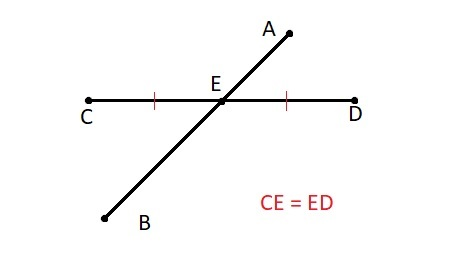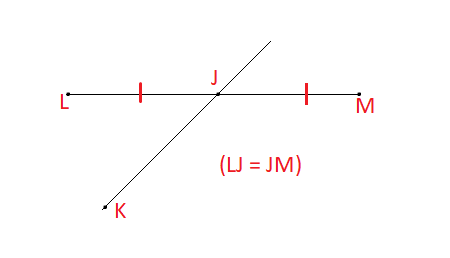# Line JK bisects LM at point J. Find JM if LJ = 23 centimeters.

## Question:

Line JK bisects LM at point J. Find JM if LJ = 23 centimeters.

## Point and Line

#### Point:

A point generally defines the location of anything. It is zero-dimensional, means it has no length, no width, and no depth.

#### Line:

A-Line is a combination of two or more than two points. The line is 1-Dimensional, which means it has the only length.

When two lines are meeting at a point that is known as an intersection point. Two straight lines are meet each other at a single point, that is the intersection point.

Line Bisector means to divide a line in two equal parts by another line.

For Example, Line AB bisects the line CD at point E, then{eq}\displaystyle CE = ED {/eq}

Given:-

The line JK Bisect the line LM at the point J.

And {eq}\displaystyle LJ = 23 {/eq} cm.

The above data we can see in the picture:So we can write that

{eq}\displaystyle JM = LJ {/eq}

And LJ = 23 cm

So

{eq}\displaystyle JM = 23 {/eq} cm.